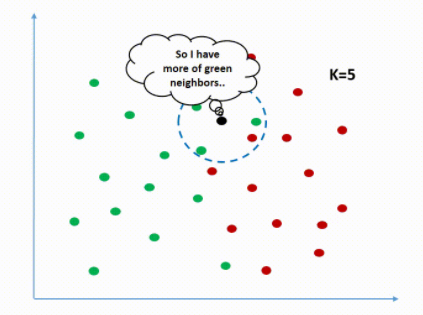# Machine Learning Classification Algorithms with Codes

Hello everyone, today I will talk about Classification Algorithms. You can find my article on the difference between Regression and Classification here.

We said that we use regression for predicting numerical data such as price prediction, and classification for problems where there is no continuous variable such as labeling, yes or no. I have to say that some algorithms can be applied and work well for both regression and classification (such as K-NN, SVM, Decision Tree and Random Forest). Today’s Topics:

• Naive Bayes Classifier (Probabilistic Based)
• K-Nearest Neighbors Classifier (Group Based)
• Logistic Regression (Maximum Entropy Based)
• Decision Tree Classifier (Tree Based)
• Random Forest Classifier (Ensemble Based-Bagging)
• Gradient Boost Classifier (Ensemble Based-Boosting)
• Support Vector Machine (SVM)

## Naive Bayes Classifier

The Naive Bayes algorithm is a classification technique based on Bayes’ Theorem with an assumption of independence among predictors. In simple terms, a Naive Bayes classifier assumes that the presence of a particular feature in a class is unrelated to the presence of any other feature.

P(A|B): The probability that event A will occur when event B occurs.

In this dataset, we will predict whether employees will be hired or not.

We divide the data into test and train. We use train to train the data, and we use the test to test our prediction.

It learns from the data with the fit function and makes predictions with the predict function.

Accuracy Score: It is the percentage of values classified as correct.

Precision Score: It shows how many of the values we guess positively are actually positive.

Recall Score: It shows “How many of the true positives have been correctly defined?”.

F1 Score: It is the harmonic mean of the Precision and Recall values. If both Precision and Recall values are important for the problem, the F1 score will also become important.

The numbers in the confusion matrix are the number of TN, FP, FN, TP values in the data. Here our probability of

Accuracy: (TP + TN) / (TN + FP + FN + TP)
Precision: TP / (FP + TP)
Sensitivity: TP / (TP + FN)
Specifity: TN / (TN + FP)

Let’s calculate the accuracy according to confusion matrix:

(233+15) / (233+16+38+15) = 0.8211920529801

## K-Nearest Neighbors ClassifierIt is included in the green class because it has more of its green neighbors.

KNN makes predictions according to the class density of the nearest neighbors of the vector formed by the independent variables of the value to be predicted. The distance of the point to be predicted to other points is calculated. The Minkowski distance calculation function is used for this. (K: We tell you how many nearest neighbors will be calculated.)

In order to obtain more successful results in distance-based algorithms such as KNN, the data are normalized.

## Logistic Regression

Logistic Regression tries to find the best line that separates the two classes. It is frequently used in linear classification problems. Because of linearity, it is very similar to Linear Regression.

The ROC curve plots the false positive rate versus the correct positive rate. The area under this curve is found with the function roc_auc_score (). The closer this field is to 1, the better the model is predicted. To find the ROC-AUC score:

## Decision Tree Classifer

Decision Tree is a type of supervised learning algorithm that is mostly used in classification problems. It starts with a single node and turns into a tree structure. It creates a model that predicts the value of a variable by extracting simple rules from data properties and learning those rules (just like a human).

Now, let’s predict the species of flowers in the Iris data set.

## Random Forest Classifier (Bagging)

In Bagging, each collection of subset data is used to train their decision trees. As a result, we end up with an ensemble of different models. Majority of the votes predictions from different trees are used which is more robust than a single decision tree.

Gradient Boosting= Gradient Descent+Boosting. It uses gradient descent algorithm which can optimize any differentiable loss function. An ensemble of trees are built one by one and individual trees are summed sequentially. Next tree tries to recover the loss (difference between actual and predicted values).